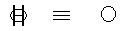磁元论（2）

2004，6公布于潜科学网站H s = eν

（2 k MQ ω = B

B为体系经线磁场，k MQ为质电结构，ω为纬线运转特性。子无磁不聚，称为磁子。物质原理描述的磁子等价于E线旋转一周：正面右旋形成正磁子，磁矩与自旋方向相同；反面左旋形成反磁子，磁矩与自旋方向相反。正反磁子称为正反物质。

▽×H = ? D/ ?t (经线)磁场方程

▽×E = ? B/ ?t (纬线)电场方程

（上面公式中的问号应当是偏微分符号，下同--编辑）

μH = BεE = D

C = (εμ) 1/2

▽·(H×E) = ?ρE/ ?t (经纬)能量方程

▽·(B×D) = ?ρm/ ?t (经纬)物质方程

（上面公式中的问号应当是偏微分符号--编者）

ρE为能量密度；ρm为质量密度。物质和能量是磁电结构聚散状态：聚则为物质，散则为能量。物质方程和能量方程导出质能关系

E = m C2

f + fˊ ≡ 0

hν = f r

h为能量子，ν为量子频率，r为空间位移矢量。能量子h以量子频率ν均匀振荡，产生量子力线空间延伸。抽取时空动力要素，则理想化为牛顿力学绝对时空：时间均匀流逝，永不停息；空间均匀分布，无限延伸。

B = kmq ω

F = m a

hν = f r

Cf = νr

eν = H s

C H = νs

（2 k MQ ω = B

h ω/2π = μQ B

μQ = (Q/2M)h/2π

μQ即为磁子，磁子振动为声，称为磁声，hω为声量子。Q/M取值“电子”荷质比，h取值普朗克常数，则μQ即玻尔磁子，描述原子体系的结构。

(dΦ/dt) = Q/4πεr

dA/dt = Q/4πεrB

k QM (dΦ/dt) = GM / r

2 r 3/T2 = GM